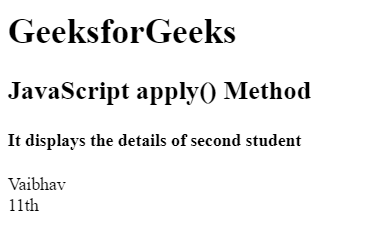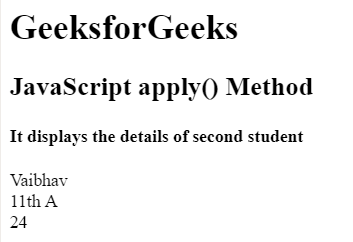# JavaScript | Function Apply

The apply() method is used to write methods, which can be used on different objects. It is different from the function call() because it takes arguments as an array.

Syntax:

`apply()`

Return Value: It returns the method values of a given function.

Example 1: This example illustrates the apply() function without arguments.

 ` ` `<``html``> ` ` `  `<``head``> ` `    ``<``title``> ` `        ``JavaScript apply() Method ` `        ``without argument ` `    `` ` ` ` ` `  `<``body``> ` `    ``<``h1``>GeeksforGeeks ` ` `  `    ``<``h2``> ` `        ``JavaScript apply() Method ` `    `` ` `     `  `    ``<``h4``> ` `        ``It displays the details of second student ` `    `` ` ` `  `    ``<``p` `id``=``"GFG"``> ` `     `  `    ``` `    ``<``script``> ` `        ``var student = { ` `            ``details: function() { ` `                ``return this.name + "<``br``>" + this.class; ` `            ``} ` `        ``} ` `        ``var stud1 = { ` `            ``name:"Dinesh", ` `            ``class: "11th", ` `        ``} ` `        ``var stud2 = { ` `            ``name:"Vaibhav", ` `            ``class: "11th", ` `        ``} ` `         `  `        ``var x = student.details.apply(stud2);  ` `        ``document.getElementById("GFG").innerHTML = x;  ` `    `` ` ` ` ` `  `                     `

Output:Example 2: This example illustrates the apply() function with arguments.

 ` ` `<``html``> ` ` `  `<``head``> ` `    ``<``title``> ` `        ``JavaScript apply() Method ` `        ``with argument ` `    `` ` ` ` ` `  `<``body``> ` `    ``<``h1``>GeeksforGeeks ` ` `  `    ``<``h2``> ` `        ``JavaScript apply() Method ` `    `` ` `     `  `    ``<``h4``> ` `        ``It displays the details of second student ` `    `` ` ` `  `    ``<``p` `id``=``"GFG"``> ` `     `  `    ``` `    ``<``script``> ` `        ``var student = { ` `            ``details: function(section, rollnum) { ` `                ``return this.name + "<``br``>" + this.class ` `                    ``+ " " + section + "<``br``>" + rollnum; ` `            ``} ` `        ``} ` `        ``var stud1 = { ` `            ``name:"Dinesh", ` `            ``class: "11th", ` `        ``} ` `        ``var stud2 = { ` `            ``name:"Vaibhav", ` `            ``class: "11th", ` `        ``} ` `         `  `        ``var x = student.details.apply(stud2, ["A", "24"]);  ` `        ``document.getElementById("GFG").innerHTML = x;  ` `    `` ` ` ` ` `  `                     `

Output:My Personal Notes arrow_drop_upCheck out this Author's contributed articles.

If you like GeeksforGeeks and would like to contribute, you can also write an article using contribute.geeksforgeeks.org or mail your article to contribute@geeksforgeeks.org. See your article appearing on the GeeksforGeeks main page and help other Geeks.

Please Improve this article if you find anything incorrect by clicking on the "Improve Article" button below.

Article Tags :

Be the First to upvote.

Please write to us at contribute@geeksforgeeks.org to report any issue with the above content.